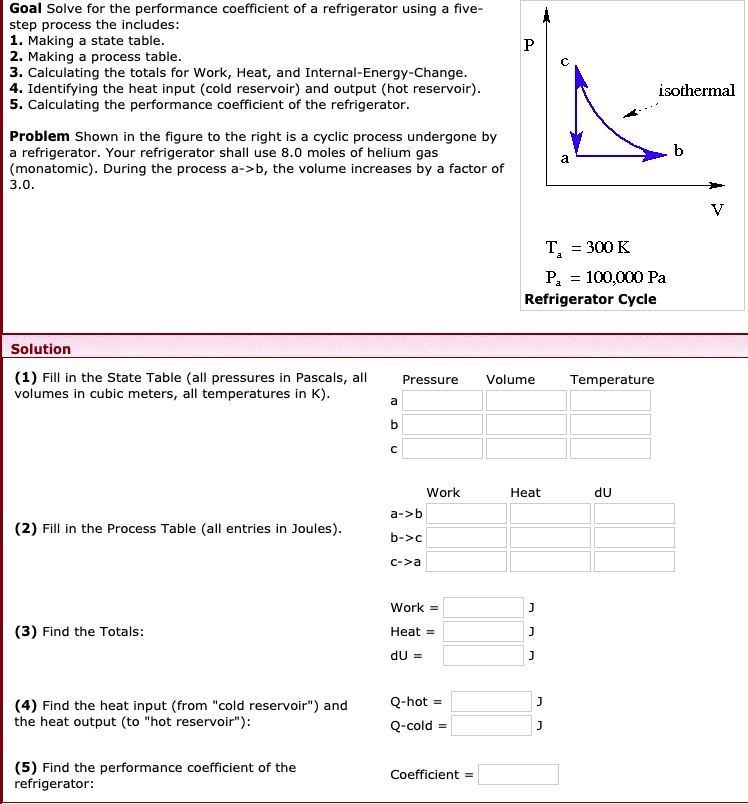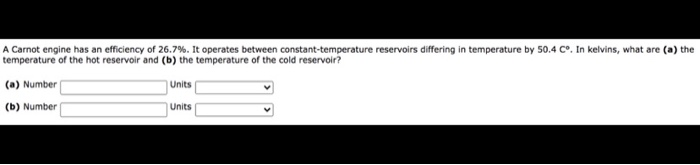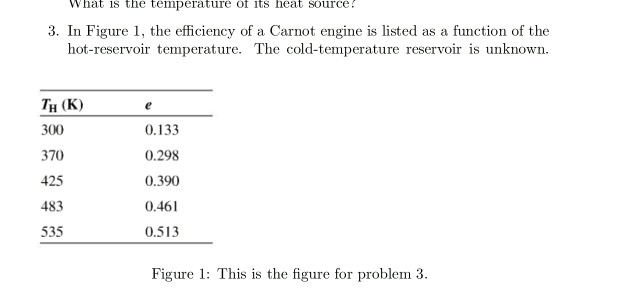# A Carnot refrigerator has a coefficient of performance of KC=4. If the temperature of the hot...

A Carnot refrigerator has a coefficient of performance of KC=4. If the temperature of the hot reservoir is TH=400K, what is the temperature of the cold temperature reservoir, TC?

This Homework Help Question: "A Carnot refrigerator has a coefficient of performance of KC=4. If the temperature of the hot..." No answers yet.

We need 10 more requests to produce the answer to this homework help question. Share with your friends to get the answer faster!

0 /10 have requested the answer to this homework help question.

Once 10 people have made a request, the answer to this question will be available in 1-2 days.
All students who have requested the answer will be notified once they are available.
##### Add Answer to: A Carnot refrigerator has a coefficient of performance of KC=4. If the temperature of the hot...
Similar Homework Help Questions
• ### Goal Solve for the performance coefficient of a refrigerator using a five- step process the includes:...Goal Solve for the performance coefficient of a refrigerator using a five- step process the includes: 1. Making a state table. 2. Making a process table. 3. Calculating the totals for Work, Heat, and Internal-Energy-Change. 4. Identifying the heat input (cold reservoir) and output (hot reservoir). 5. Calculating the performance coefficient of the refrigerator. isothermal Problem Shown in the figure to the right is a cyclic process undergone by a refrigerator. Your refrigerator shall use 8.0 moles of helium gas...

• ### Suppose you want to operate an ideal refrigerator that has a cold temperature of -11°C, and...

Suppose you want to operate an ideal refrigerator that has a cold temperature of -11°C, and you would like it to have a coefficient of performance of 8.5. What is the temperature, in degrees Celsius, of the hot reservoir for such a refrigerator?

• ### A Carnot engine has an efficiency of 0.45. The Kelvin temperature of its hot reservoir is...

A Carnot engine has an efficiency of 0.45. The Kelvin temperature of its hot reservoir is quadrupled, and the Kelvin temperature of its cold reservoir is doubled. What is the efficiency that results from these changes?

• ### A refrigerator has a coefficient of performance equal to 4.4. If the refrigerator absorbs 203.4 J...

A refrigerator has a coefficient of performance equal to 4.4. If the refrigerator absorbs 203.4 J of thermal energy from a cold reservoir in each cycle, find the work done on the system each cycle (in J). Enter a number with one digit behind the decimal point.

• ### coefficient of performance

A refrigerator has a coefficient of performance of 1.50, and it extracts 6.95 J of heat from the cold reservoir during each cycle. (a) How much work is done on the gasin each cycle? (b) How much heat is exhausted into the hot reservoir in each cycle?

• ### A Carnot engine has an efficiency of 0.445, and the temperature of its cold reservoir is...

A Carnot engine has an efficiency of 0.445, and the temperature of its cold reservoir is 434 K. (a) Determine the temperature of its hot reservoir. (b) If 5610 J of heat is rejected to the cold reservoir, what amount of heat is put into the engine?

• ### A Carnot engine has an efficiency of 26.7%. It operates between constant-temperature reservoirs differing in temperature...A Carnot engine has an efficiency of 26.7%. It operates between constant-temperature reservoirs differing in temperature by 50.4 Cº. In kelvins, what are (a) the temperature of the hot reservoir and (b) the temperature of the cold reservoir? (a) Number Units (b) Number Units

• ### 1s the temperature of 3. In Figure 1, the efficiency of a Carnot engine is listed...1s the temperature of 3. In Figure 1, the efficiency of a Carnot engine is listed as a function of the hot-reservoir temperature. The cold-temperature reservoir is unknown TH (K) 300 370 425 483 535 0.133 0.298 0.390 0.461 0.513 Figure 1: This is the figure for problem 3

• ### A refrigerator with a coefficient of performance of 1.80 absorbs 3.15×104 J of heat from the...

A refrigerator with a coefficient of performance of 1.80 absorbs 3.15×104 J of heat from the low-temperature reservoir during each cycle. How much mechanical work is required to operate the refrigerator for a cycle? How much heat does the refrigerator discard to the high-temperature reservoir during each cycle?

• ### A Carnot engine operates with an efficiency of 29% when the temperature of its cold reservoir...

A Carnot engine operates with an efficiency of 29% when the temperature of its cold reservoir is 295 K. Assuming that the temperature of the hot reservoir remains the same, what must be the temperature of the cold reservoir in order to increase the efficiency to 33%?

Free Homework App